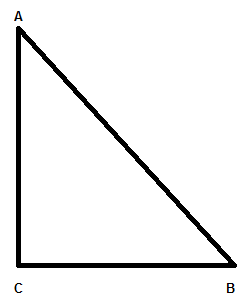Courses
Courses for Kids
Free study material
Free LIVE classes
MoreLIVE
Join Vedantu’s FREE Mastercalss

# In the given figure, ABC is an isosceles right triangle, right-angled at C. Prove that $A{{B}^{2}}=2A{{C}^{2}}$.Verified
360.3k+ views
Hint: Two sides of an isosceles triangle is equal. Pythagoras theorem can be given as ${{\left( \text{hypotaneous} \right)}^{\text{2}}}\text{=}{{\left( \text{base} \right)}^{\text{2}}}\text{+}{{\left( \text{perpendicular} \right)}^{\text{2}}}$. Using these two things the desired result can be obtained.

We have an isosceles triangle ABC with$\angle C={{90}^{o}}$ and hence sides AC and BC are equal to each other, as in isosceles triangle two sides are equal. Hence, diagram of $\Delta ABC$can be represented asWhere AC = BC and $\angle C={{90}^{o}}$
Now, we need to prove the relation given as
$A{{B}^{2}}=2A{{C}^{2}}$ ………………….. (i)
So, let us calculate the value of $A{{B}^{2}}$in terms of $A{{C}^{2}}$to prove the above equation (i).
As we know any right angle triangle will follow the Pythagoras property which can be given as
${{\left( \text{hypotaneous} \right)}^{\text{2}}}\text{=}{{\left( \text{base} \right)}^{\text{2}}}\text{+}{{\left( \text{perpendicular} \right)}^{\text{2}}}$………… (iii)
Hence, we can write the equation (iii) in terms of sides of $\Delta ABC$ as
${{\left( AB \right)}^{2}}={{\left( BC \right)}^{2}}+{{\left( AC \right)}^{2}}$…………… (iv)
Now, as the given triangle is isosceles, therefore AC=BC from the figure; and hence, we can replace BC by AC in the equation (iv), so, we get
\begin{align} & {{\left( AB \right)}^{2}}={{\left( AC \right)}^{2}}+{{\left( AC \right)}^{2}} \\ & \Rightarrow {{\left( AB \right)}^{2}}=2{{\left( AC \right)}^{2}} \\ \end{align}
Hence, equation (i) or the given relation is proved.

Note:
One can go wrong with the Pythagoras theorem. One may put the value of base or perpendicular in place of hypotenuse or vice-versa may also happen. Hence, be clear with the terms of the Pythagoras theorem.
One can get angles A and B as ${{45}^{o}}$. As both are equal and summation of all the angles of the triangle is ${{180}^{0}}$. Now, take $\sin {{45}^{o}}$in the triangle with respect to angle B.
Hence $\sin B=\sin {{45}^{o}}=\dfrac{AC}{AB}$
Now, put $\sin {{45}^{o}}=\dfrac{1}{\sqrt{2}}$ and square both sides.
We will get the same result as given in the question.
Last updated date: 21st Sep 2023
Total views: 360.3k
Views today: 4.60k